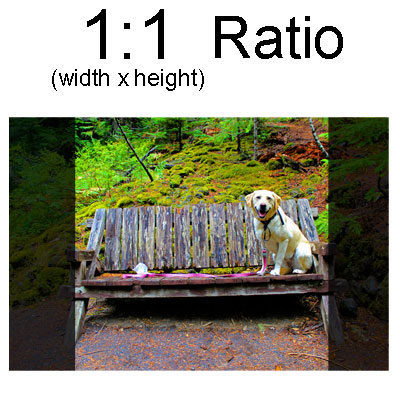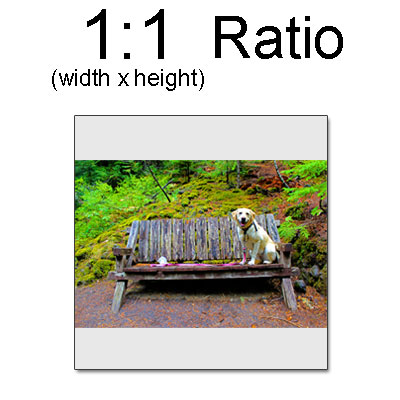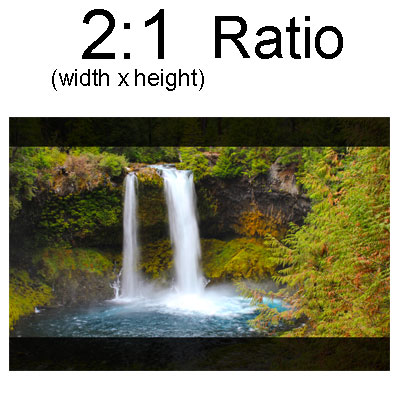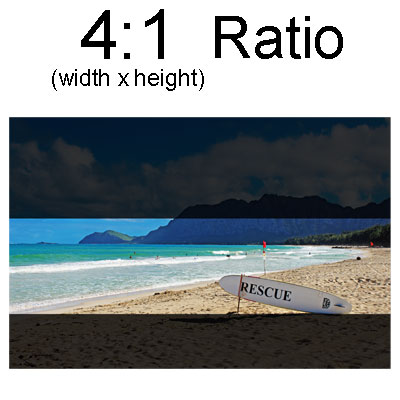# Image Ratio for Printing

## What is Aspect ratio?

Aspect ratio refers to the relationship between the width and height of an image. The number before the colon represents the width of the image and the number after is the height. Both numbers represent a relationship, not a specific measurement.

## How does Aspect Ratio affect printing?

The aspect ratio will never affect the quality of a print, only how it is cropped. For example, if an image is uploaded with an aspect ratio of 3:2 but dimensions of 5 inches x 5 inches are selected then the image will be cropped to square, or a white boarder will be left on the top and bottom. This occurs because the file ratio of 3:2 was not equal to the selected ratio of 1:1.## How does aspect ratio affect the size of a print?

Because aspect ratio represents the width to height relationship, it doesn’t limit or restrict the overall size of a print.
Typically we like to ask customers how wide they would like their print. By selecting a width we can determine height using the image aspect ratio of the original file.
For example: If you have an image with an aspect ratio of 3:2 and you want a print that is 12 inches wide, the height will be 66% (or 2/3) the size of the width. 12 *.66 = ~8 inches.

##You need to choose options for your item

x